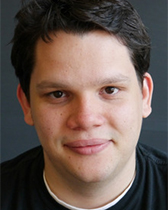# DEPARTMENT OF MATHEMATICS

## Antonio Auffinger

PhD, New York University### Biography

Tuca's research is on probability theory (sub-additive processes, spin glasses, gaussian fields and random matrices) and its connections with other domains of mathematics (partial differential equations, dynamical systems), physics (statistical mechanics of disordered media), and theoretical biology (ecology). His main interest is in the complexity of such disordered systems and the universal aspects of their long time behavior.

### Selected Publications

1. A. Auffinger, W.-K. Chen. The Parisi formula has a unique minimizer. To appear in Comm. in Math. Phys.
2. A. Auffinger, M. Damron. A simplified proof of the relation between scaling exponents in first-passage percolation. Annals of Probability 2014, Vol. 42, No. 3, 1197-1211.
3. A. Auffinger, G. Ben Arous. Complexity of random smooth functions of many variables. Annals of Probability 2013, Vol. 41, No. 6, 4214-4247.